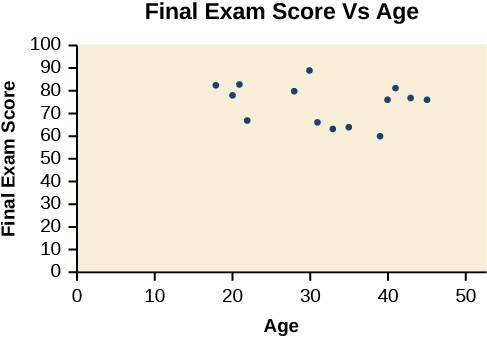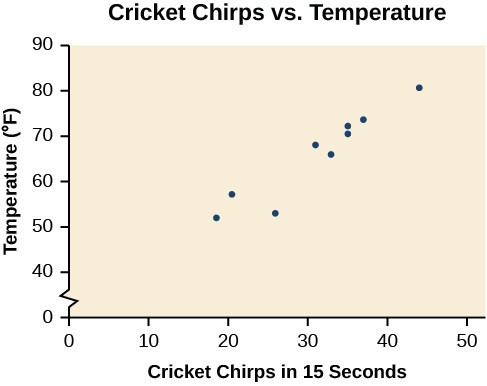## Draw and interpret scatter plots

A scatter plot is a graph of plotted points that may show a relationship between two sets of data. If the relationship is from a linear model, or a model that is nearly linear, the professor can draw conclusions using his knowledge of linear functions. Below is a sample scatter plot.Figure 1. A scatter plot of age and final exam score variables

Notice this scatter plot does not indicate a linear relationship. The points do not appear to follow a trend. In other words, there does not appear to be a relationship between the age of the student and the score on the final exam.

### Example 1: Using a Scatter Plot to Investigate Cricket Chirps

The table below shows the number of cricket chirps in 15 seconds, for several different air temperatures, in degrees Fahrenheit. Plot this data, and determine whether the data appears to be linearly related.

 Chirps 44 35 20.4 33 31 35 18.5 37 26 Temperature 80.5 70.5 57 66 68 72 52 73.5 53

### Solution

Plotting this data suggests that there may be a trend. We can see from the trend in the data that the number of chirps increases as the temperature increases. The trend appears to be roughly linear, though certainly not perfectly so.Figure 2

1. Selected data from http://classic.globe.gov/fsl/scientistsblog/2007/10/. Retrieved Aug 3, 2010